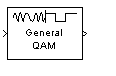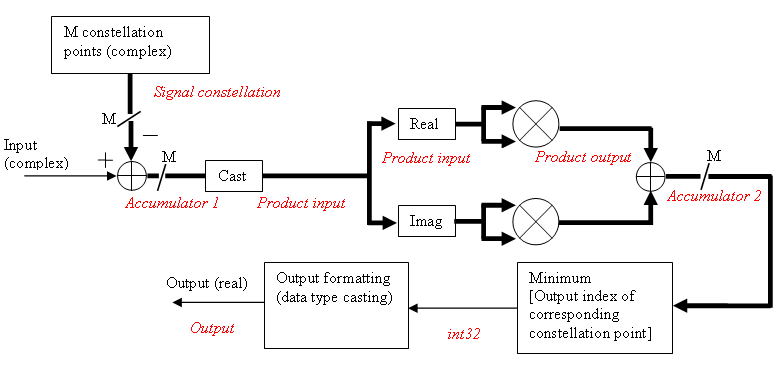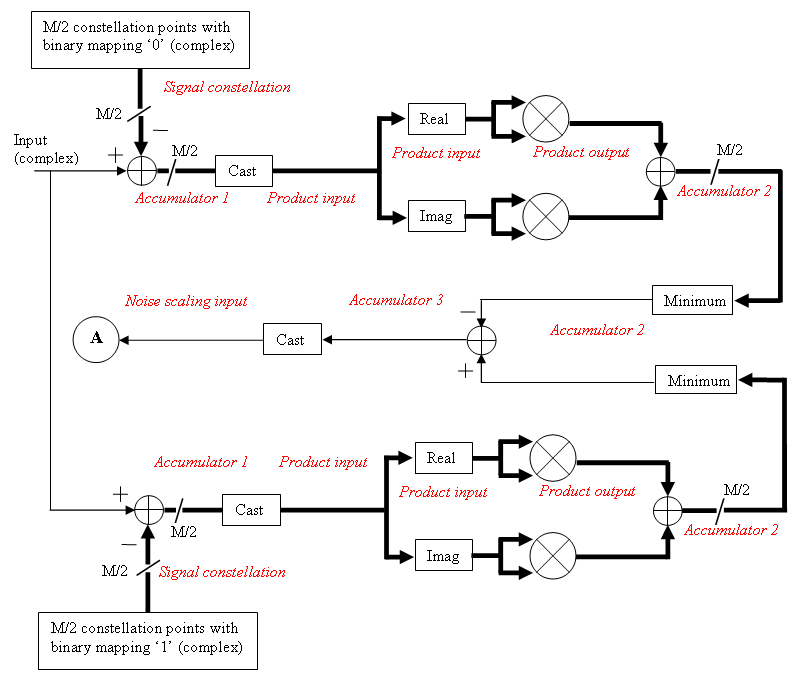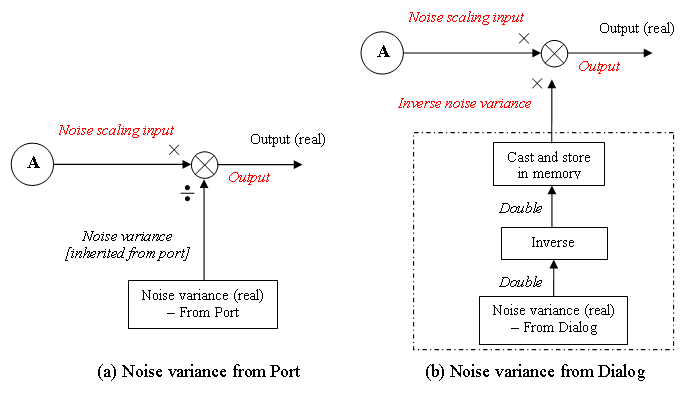# General QAM Demodulator Baseband

Demodulate QAM-modulated data

## Library

AM, in Digital Baseband sublibrary of Modulation

•## Description

The General QAM Demodulator Baseband block demodulates a signal that was modulated using quadrature amplitude modulation. The input is a baseband representation of the modulated signal.

The input must be a discrete-time complex signal. The Signal constellation parameter defines the constellation by listing its points in a length-M vector of complex numbers. The block maps the mth point in the Signal constellation vector to the integer m-1.

This block accepts a scalar or column vector input signal. For information about the data types each block port supports, see the Supported Data Types table on this page.

## Parameters

Signal constellation

A real or complex vector that lists the constellation points.

Output type

Determines whether the block produces integers or binary representations of integers.

If you set this parameter to `Integer`, the block produces integers.

If you set this parameter to `Bit`, the block produces a group of K bits, called a binary word, for each symbol, when Decision type is set to `Hard decision`. If Decision type is set to `Log-likelihood ratio` or ```Approximate log-likelihood ratio```, the block outputs bitwise LLR and approximate LLR, respectively.

Decision type

Specifies the use of hard decision, LLR, or approximate LLR during demodulation. For more information, see Hard- vs. Soft-Decision Demodulation.

To enable this parameter, set Output type to `Bit`.

Variance source

When you set this parameter to `Dialog`, you can then specify the noise variance in the Variance parameter. When you set this option to `Port`, a port appears on the block through which the noise variance can be input.

To enable this parameter, set Decision type to `Approximate log-likelihood ratio` or `Log-likelihood ratio`.

Variance

This parameter appears when the Variance source is set to `Dialog` and specifies the noise variance in the input signal. This parameter is tunable in normal mode, Accelerator mode, and Rapid Accelerator mode. This parameter is nontunable for fixed-point inputs.

If you use the Simulink® Coder™ rapid simulation (RSIM) target to build an RSIM executable, then you can tune the parameter without recompiling the model. This is useful for Monte Carlo simulations in which you run the simulation multiple times (perhaps on multiple computers) with different amounts of noise.

The exact LLR algorithm computes exponentials using finite precision arithmetic. For computations involving very large positive or negative magnitudes, the exact LLR algorithm yields:

• `Inf` or `-Inf` if the noise variance is a very large value

• `NaN` if the noise variance and signal power are both very small values

The approximate LLR algorithm does not compute exponentials. You can avoid `Inf`, `-Inf`, and `NaN` results by using the approximate LLR algorithm.

## Fixed-Point Signal Flow Diagrams

Fixed-Point Signal Flow Diagram for Hard Decision ModeNote

In the figure above, M represents the size of the Signal constellation.

The general QAM Demodulator Baseband block supports fixed-point operations for computing hard decision (Output type set to `Bit` and Decision type is set to `Hard decision`) and approximate LLR (Output type is set to `Bit` and Decision type is set to `Approximate log-likelihood ratio`) output values. The input values must have fixed-point data type for fixed-point operations.

Note

Fixed-point operations are NOT yet supported for exact LLR output values.

Fixed-Point Signal Flow Diagram for Approximate LLR ModeNote

In the figure above, M represents the size of the Signal constellation.

Fixed-Point Signal Flow Diagram for Approximate LLR Mode: Noise Variance Operation ModesNote

If Variance source is set to `Dialog`, the block performs the operations shown inside the dotted line once during initialization. The block also performs these operations if the Variance value changes during simulation.

## Data Types Attributes

Output

The block supports the following Output options:

When you set the parameter to `Inherit via internal rule` (default setting), the block inherits the output data type from the input port. The output data type is the same as the input data type if the input is of type `single` or `double`.

For integer outputs, you can set this block's output to ```Inherit via internal rule``` (default setting), `Smallest unsigned integer`, `int8`, `uint8`, `int16`, `uint16`, `int32`, `uint32`, `single`, and `double`.

For bit outputs, when you set Decision type to `Hard decision`, you can set the output to ```Inherit via internal rule```, ```Smallest unsigned integer```, `int8`, `uint8`, `int16`, `uint16`, `int32`, `uint32`, `boolean`, `single`, or `double`.

When you set Decision type to ```Hard decision``` or ```Approximate log-likelihood ratio``` and the input is a floating point data type, then the output inherits its data type from the input. For example, if the input is of data type `double`, the output is also of data type `double`. When you set Decision type to `Hard decision` or ```Approximate log-likelihood ratio```, and the input is a fixed-point signal, the Output parameter, located in the Fixed-Point algorithm parameters region of the Data-Type tab, specifies the output data type.

When you set the parameter to `Smallest unsigned integer`, the output data type is selected based on the settings used in the Hardware Implementation pane of the Configuration Parameters dialog box. If you select `ASIC/FPGA` in the Hardware Implementation pane, the output data type is the ideal minimum size, i.e., `ufix(1)` for bit outputs, and $ufix\left(⌈{\mathrm{log}}_{2}M⌉\right)$ for integer outputs. For all other choices, the Output data type is an unsigned integer with the smallest available word length large enough to fit the ideal minimum size, usually corresponding to the size of a char (e.g., `uint8`).

Rounding Mode Parameter

Use this parameter to specify the rounding method to be used when the result of a fixed-point calculation does not map exactly to a number representable by the data type and scaling storing the result.

For more information, see Rounding Modes or Rounding Mode: Simplest (Fixed-Point Designer).

Saturate on integer overflow

Use this parameter to specify the method to be used if the magnitude of a fixed-point calculation result does not fit into the range of the data type and scaling that stores the result:

• Saturate represents positive overflows as the largest positive number in the range being used, and negative overflows as the largest negative number in the range being used.

• Wrap uses modulo arithmetic to cast an overflow back into the representable range of the data type. See Modulo Arithmetic (Fixed-Point Designer) for more information.

For more information, see the Saturate on integer overflow parameter subsection of Specify Fixed-Point Attributes for Blocks.

Signal constellation

Use this parameter to define the data type of the Signal constellation parameter.

• When you select ```Same word length as input``` the word length of the Signal constellation parameter matches that of the input to the block. The fraction length is computed to provide the best precision for given signal constellation values.

• Select ```Specify word length```, to enable Word Length parameter, and then you can modify the word length. The fraction length is computed to provide the best precision for given signal constellation values.

Accumulator 1

Use this parameter to specify the data type for Accumulator 1:

• When you select ```Inherit via internal rule```, the block automatically calculates the output word and fraction lengths. For more information, see Inherit via Internal Rule.

• When you select ```Binary point scaling```, you can enter the word length and the fraction length of Accumulator 1, in bits.

Product Input

Use this parameter to specify the data type for Product input.

• When you select ```Same as accumulator 1```, the Product Input characteristics match those of Accumulator 1.

• When you select ```Binary point scaling``` you can enter the word length and the fraction length of Product input, in bits.

Product Output

Use this parameter to select the data type for Product output.

• When you select``` Inherit via internal rule```, the block automatically calculates the output signal type. For more information, see Inherit via Internal Rule.

• When you select ```Binary point scaling``` enter the word length and the fraction length for Product output, in bits.

Accumulator 2

Use this parameter to specify the data type for Accumulator 2:

• When you select ```Inherit via internal rule```, the block automatically calculates the accumulator data type. The internal rule calculates the ideal, full-precision word length and fraction length as follows:

WLideal accumulator 2 = WLinput to accumulator 2

FLideal accumulator 2 = FL input to accumulator 2

After the full-precision result is calculated, your particular hardware may still affect the final word and fraction lengths set by the internal rule. For more information, see The Effect of the Hardware Implementation Pane on the Internal Rule.

The internal rule always sets the sign of data-type to `Unsigned` .

• When you select ```Binary point scaling```, you are able to enter the word length and the fraction length of Accumulator 2, in bits.

The settings for the following fixed-point parameters only apply when you set Decision type to ```Approximate log-likelihood ratio```.

Accumulator 3

When you select ```Inherit via internal rule```, the block automatically calculates the accumulator data type. The internal rule first calculates ideal, full-precision word length and fraction length as follows:

WLideal accumulator 3 = WLinput to accumulator 3 + 1

FL ideal accumulator 3 = FL input to accumulator 3.

After the full-precision result is calculated, your particular hardware may still affect the final word and fraction lengths set by the internal rule. For more information, see The Effect of the Hardware Implementation Pane on the Internal Rule.

The internal rule always sets the sign of data-type to `Signed`.

Noise scaling input
• When you select ```Same as accumulator 3```, the Noise scaling input characteristics match those of Accumulator 3.

• When you select ```Binary point scaling``` you are able to enter the word length and the fraction length of Noise scaling input, in bits.

Inverse noise variance

To enable this parameter, set Variance source to `Dialog`. This parameter appears in the tab.

• When you select ```Same word length as input``` the word length of the Inverse noise variance parameter matches that of the input to the block. The fraction length is computed to provide the best precision for a given inverse noise variance value.

• When you select ```Specify word length```, the Word Length parameter appears, and then you can modify the word length. The fraction length is computed to provide the best precision for a given inverse noise variance value.

Output

When you select ```Inherit via internal rule``` , the Output data type is automatically set for you.

If you set the Variance source parameter to `Dialog`, the output is a result of product operation as shown in the Noise Variance Operation Modes Signal Flow Diagram Fixed-Point Signal Flow Diagram for Approximate LLR Mode: Noise Variance Operation Modes. In this case, it follows the internal rule for Product data types specified in the Inherit via Internal Rule topic.

If the Variance source parameter is set to `Port`, the output is a result of division operation as shown in the signal flow diagram. In this case, the internal rule calculates the ideal, full-precision word length and fraction length as follows:

WL output = max(WL Noise scaling input, WL Noise variance)

FL output = FL Noise scaling input (dividend)FL Noise variance (divisor) .

After the full-precision result is calculated, your particular hardware may still affect the final word and fraction lengths set by the internal rule. For more information, see The Effect of the Hardware Implementation Pane on the Internal Rule.

The internal rule for Output always sets the sign of data-type to `Signed`.

For additional information about the parameters pertaining to fixed-point applications, see Specify Fixed-Point Attributes for Blocks.

## Supported Data Types

PortSupported Data Types

Input

• Double-precision floating point

• Single-precision floating point

• Signed fixed–point when Output type is `Integer` or Output type is `Bit` and Decision type is either `Hard decision` or ```Approximate log-likelihood ratio```

Var

• Double-precision floating point

• Single-precision floating point

Output

• Double-precision floating point

• Single-precision floating point

• Boolean when Output type is `Bit` and Decision type is `Hard decision`.

• 8-, 16-, and 32-bit signed integers when Output type is `Integer` or Output type is `Bit` and Decision type is `Hard decision`

• 8-, 16-, and 32-bit unsigned integers when Output type is `Integer` or Output type is `Bit` and Decision type is `Hard decision`

• ufix(1) in ASIC/FPGA when Output type is `Bit` and Decision type is `Hard decision`

• $ufix\left(⌈{\mathrm{log}}_{2}M⌉\right)$ in ASIC/FPGA when Output type is `Integer`

• Signed fixed-point when Output type is `Bit` and Decision type is ```Approximate log-likelihood ratio```

## Pair Block

General QAM Modulator Baseband

## Version History

Introduced before R2006a